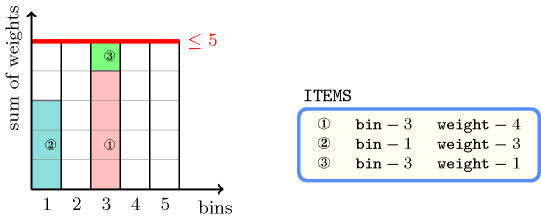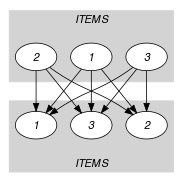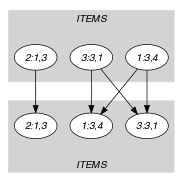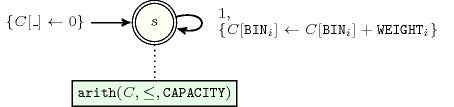## 5.53. bin_packing

Origin
Constraint

$\mathrm{𝚋𝚒𝚗}_\mathrm{𝚙𝚊𝚌𝚔𝚒𝚗𝚐}\left(\mathrm{𝙲𝙰𝙿𝙰𝙲𝙸𝚃𝚈},\mathrm{𝙸𝚃𝙴𝙼𝚂}\right)$

Arguments
 $\mathrm{𝙲𝙰𝙿𝙰𝙲𝙸𝚃𝚈}$ $\mathrm{𝚒𝚗𝚝}$ $\mathrm{𝙸𝚃𝙴𝙼𝚂}$ $\mathrm{𝚌𝚘𝚕𝚕𝚎𝚌𝚝𝚒𝚘𝚗}\left(\mathrm{𝚋𝚒𝚗}-\mathrm{𝚍𝚟𝚊𝚛},\mathrm{𝚠𝚎𝚒𝚐𝚑𝚝}-\mathrm{𝚒𝚗𝚝}\right)$
Restrictions
 $\mathrm{𝙲𝙰𝙿𝙰𝙲𝙸𝚃𝚈}\ge 0$ $\mathrm{𝚛𝚎𝚚𝚞𝚒𝚛𝚎𝚍}$$\left(\mathrm{𝙸𝚃𝙴𝙼𝚂},\left[\mathrm{𝚋𝚒𝚗},\mathrm{𝚠𝚎𝚒𝚐𝚑𝚝}\right]\right)$ $\mathrm{𝙸𝚃𝙴𝙼𝚂}.\mathrm{𝚠𝚎𝚒𝚐𝚑𝚝}\ge 0$ $\mathrm{𝙸𝚃𝙴𝙼𝚂}.\mathrm{𝚠𝚎𝚒𝚐𝚑𝚝}\le \mathrm{𝙲𝙰𝙿𝙰𝙲𝙸𝚃𝚈}$
Purpose

Given several items of the collection $\mathrm{𝙸𝚃𝙴𝙼𝚂}$ (each of them having a specific weight), and different bins of a fixed capacity, assign each item to a bin so that the total weight of the items in each bin does not exceed $\mathrm{𝙲𝙰𝙿𝙰𝙲𝙸𝚃𝚈}$.

Example
$\left(\begin{array}{c}5,〈\begin{array}{cc}\mathrm{𝚋𝚒𝚗}-3\hfill & \mathrm{𝚠𝚎𝚒𝚐𝚑𝚝}-4,\hfill \\ \mathrm{𝚋𝚒𝚗}-1\hfill & \mathrm{𝚠𝚎𝚒𝚐𝚑𝚝}-3,\hfill \\ \mathrm{𝚋𝚒𝚗}-3\hfill & \mathrm{𝚠𝚎𝚒𝚐𝚑𝚝}-1\hfill \end{array}〉\hfill \end{array}\right)$

The $\mathrm{𝚋𝚒𝚗}_\mathrm{𝚙𝚊𝚌𝚔𝚒𝚗𝚐}$ constraint holds since the sum of the height of items that are assigned to bins 1 and 3 is respectively equal to 3 and 5. The previous quantities are both less than or equal to the maximum $\mathrm{𝙲𝙰𝙿𝙰𝙲𝙸𝚃𝚈}$ 5. Figure 5.53.1 shows the solution associated with the example.

##### Figure 5.53.1. Bin-packing solution to the Example slotTypical
 $\mathrm{𝙲𝙰𝙿𝙰𝙲𝙸𝚃𝚈}>$$\mathrm{𝚖𝚊𝚡𝚟𝚊𝚕}$$\left(\mathrm{𝙸𝚃𝙴𝙼𝚂}.\mathrm{𝚠𝚎𝚒𝚐𝚑𝚝}\right)$ $\mathrm{𝙲𝙰𝙿𝙰𝙲𝙸𝚃𝚈}\le$$\mathrm{𝚜𝚞𝚖}$$\left(\mathrm{𝙸𝚃𝙴𝙼𝚂}.\mathrm{𝚠𝚎𝚒𝚐𝚑𝚝}\right)$ $|\mathrm{𝙸𝚃𝙴𝙼𝚂}|>1$ $\mathrm{𝚛𝚊𝚗𝚐𝚎}$$\left(\mathrm{𝙸𝚃𝙴𝙼𝚂}.\mathrm{𝚋𝚒𝚗}\right)>1$ $\mathrm{𝚛𝚊𝚗𝚐𝚎}$$\left(\mathrm{𝙸𝚃𝙴𝙼𝚂}.\mathrm{𝚠𝚎𝚒𝚐𝚑𝚝}\right)>1$ $\mathrm{𝙸𝚃𝙴𝙼𝚂}.\mathrm{𝚋𝚒𝚗}\ge 0$ $\mathrm{𝙸𝚃𝙴𝙼𝚂}.\mathrm{𝚠𝚎𝚒𝚐𝚑𝚝}>0$
Symmetries
• $\mathrm{𝙲𝙰𝙿𝙰𝙲𝙸𝚃𝚈}$ can be increased.

• Items of $\mathrm{𝙸𝚃𝙴𝙼𝚂}$ are permutable.

• $\mathrm{𝙸𝚃𝙴𝙼𝚂}.\mathrm{𝚠𝚎𝚒𝚐𝚑𝚝}$ can be decreased to any value $\ge 0$.

• All occurrences of two distinct values of $\mathrm{𝙸𝚃𝙴𝙼𝚂}.\mathrm{𝚋𝚒𝚗}$ can be swapped; all occurrences of a value of $\mathrm{𝙸𝚃𝙴𝙼𝚂}.\mathrm{𝚋𝚒𝚗}$ can be renamed to any unused value.

Arg. properties

Contractible wrt. $\mathrm{𝙸𝚃𝙴𝙼𝚂}$.

Remark

Note the difference with the classical bin-packing problem [MartelloToth90] where one wants to find solutions that minimise the number of bins. In our case each item may be assigned only to specific bins (i.e., the different values of the bin variable) and the goal is to find a feasible solution. This constraint can be seen as a special case of the $\mathrm{𝚌𝚞𝚖𝚞𝚕𝚊𝚝𝚒𝚟𝚎}$ constraint [AggounBeldiceanu93], where all task durations are equal to 1.

In [Shaw04] the $\mathrm{𝙲𝙰𝙿𝙰𝙲𝙸𝚃𝚈}$ parameter of the $\mathrm{𝚋𝚒𝚗}_\mathrm{𝚙𝚊𝚌𝚔𝚒𝚗𝚐}$ constraint is replaced by a collection of domain variables representing the load of each bin (i.e., the sum of the weights of the items assigned to a bin). This allows representing problems where a minimum level has to be reached in each bin.

Coffman and al. give in [CoffmanGareyJohnson84] the worst case bounds of different list algorithms for the bin packing problem (i.e., given a positive integer $\mathrm{𝙲𝙰𝙿𝙰𝙲𝙸𝚃𝚈}$ and a list $L$ of integer sizes ${\mathrm{𝚠𝚎𝚒𝚐𝚑𝚝}}_{1},{\mathrm{𝚠𝚎𝚒𝚐𝚑𝚝}}_{2},\cdots ,{\mathrm{𝚠𝚎𝚒𝚐𝚑𝚝}}_{n}$ $\left(0\le {\mathrm{𝚠𝚎𝚒𝚐𝚑𝚝}}_{i}\le \mathrm{𝙲𝙰𝙿𝙰𝙲𝙸𝚃𝚈}\right)$, what is the smallest integer $m$ such that there is a partition $L={L}_{1}\cup {L}_{2}\cup \cdots \cup {L}_{m}$ satisfying ${\sum }_{{\mathrm{𝚠𝚎𝚒𝚐𝚑𝚝}}_{i}\in {L}_{j}}{\mathrm{𝚠𝚎𝚒𝚐𝚑𝚝}}_{i}\le \mathrm{𝙲𝙰𝙿𝙰𝙲𝙸𝚃𝚈}$ for all $j\in \left[1,m\right]$?).

Algorithm

Initial filtering algorithms are described in [MullerHannemannStilleWeihe03a], [MullerHannemannStilleWeihe03b], [MullerHannemannStilleWeihe03c], [MullerHannemannStilleWeihe03d], [Shaw04]. More recently, linear continuous relaxations based on the graph associated with the dynamic programming approach for knapsack by Trick [Trick03], and on the more compact model introduced by Carvalho [Carvalho99], [Carvalho02] are presented in [CambazardSullivan10].

Systems

generalisation: $\mathrm{𝚋𝚒𝚗}_\mathrm{𝚙𝚊𝚌𝚔𝚒𝚗𝚐}_\mathrm{𝚌𝚊𝚙𝚊}$ (fixed overall capacity replaced by non-fixed capacity), $\mathrm{𝚌𝚞𝚖𝚞𝚕𝚊𝚝𝚒𝚟𝚎}$ (task of $\mathrm{𝚍𝚞𝚛𝚊𝚝𝚒𝚘𝚗}$ 1 replaced by task of given $\mathrm{𝚍𝚞𝚛𝚊𝚝𝚒𝚘𝚗}$), $\mathrm{𝚌𝚞𝚖𝚞𝚕𝚊𝚝𝚒𝚟𝚎}_\mathrm{𝚝𝚠𝚘}_𝚍$ ($\mathrm{𝚝𝚊𝚜𝚔}$ of $\mathrm{𝚍𝚞𝚛𝚊𝚝𝚒𝚘𝚗}$ 1 replaced by $\mathrm{𝚜𝚚𝚞𝚊𝚛𝚎}$ of size 1 with a $\mathrm{𝚑𝚎𝚒𝚐𝚑𝚝}$), $\mathrm{𝚒𝚗𝚍𝚎𝚡𝚎𝚍}_\mathrm{𝚜𝚞𝚖}$ (negative contribution also allowed, fixed capacity replaced by a set of variables).

Keywords
Cond. implications

$\mathrm{𝚋𝚒𝚗}_\mathrm{𝚙𝚊𝚌𝚔𝚒𝚗𝚐}\left(\mathrm{𝙲𝙰𝙿𝙰𝙲𝙸𝚃𝚈},\mathrm{𝙸𝚃𝙴𝙼𝚂}\right)$

with  $\mathrm{𝙲𝙰𝙿𝙰𝙲𝙸𝚃𝚈}\ge |\mathrm{𝙸𝚃𝙴𝙼𝚂}|$

implies $\mathrm{𝚊𝚝𝚖𝚘𝚜𝚝}_\mathrm{𝚗𝚟𝚎𝚌𝚝𝚘𝚛}$$\left(\mathrm{𝙽𝚅𝙴𝙲}:\mathrm{𝙲𝙰𝙿𝙰𝙲𝙸𝚃𝚈},\mathrm{𝚅𝙴𝙲𝚃𝙾𝚁𝚂}:\mathrm{𝙸𝚃𝙴𝙼𝚂}\right)$.

Arc input(s)

$\mathrm{𝙸𝚃𝙴𝙼𝚂}$ $\mathrm{𝙸𝚃𝙴𝙼𝚂}$

Arc generator
$\mathrm{𝑃𝑅𝑂𝐷𝑈𝐶𝑇}$$↦\mathrm{𝚌𝚘𝚕𝚕𝚎𝚌𝚝𝚒𝚘𝚗}\left(\mathrm{𝚒𝚝𝚎𝚖𝚜}\mathtt{1},\mathrm{𝚒𝚝𝚎𝚖𝚜}\mathtt{2}\right)$

Arc arity
Arc constraint(s)
$\mathrm{𝚒𝚝𝚎𝚖𝚜}\mathtt{1}.\mathrm{𝚋𝚒𝚗}=\mathrm{𝚒𝚝𝚎𝚖𝚜}\mathtt{2}.\mathrm{𝚋𝚒𝚗}$
Graph class
 $•$$\mathrm{𝙰𝙲𝚈𝙲𝙻𝙸𝙲}$ $•$$\mathrm{𝙱𝙸𝙿𝙰𝚁𝚃𝙸𝚃𝙴}$ $•$$\mathrm{𝙽𝙾}_\mathrm{𝙻𝙾𝙾𝙿}$

Sets
$\begin{array}{c}\mathrm{𝖲𝖴𝖢𝖢}↦\hfill \\ \left[\begin{array}{c}\mathrm{𝚜𝚘𝚞𝚛𝚌𝚎},\hfill \\ \mathrm{𝚟𝚊𝚛𝚒𝚊𝚋𝚕𝚎𝚜}-\mathrm{𝚌𝚘𝚕}\left(\begin{array}{c}\mathrm{𝚅𝙰𝚁𝙸𝙰𝙱𝙻𝙴𝚂}-\mathrm{𝚌𝚘𝚕𝚕𝚎𝚌𝚝𝚒𝚘𝚗}\left(\mathrm{𝚟𝚊𝚛}-\mathrm{𝚍𝚟𝚊𝚛}\right),\hfill \\ \mathrm{𝚒𝚝𝚎𝚖}\left(\mathrm{𝚟𝚊𝚛}-\mathrm{𝙸𝚃𝙴𝙼𝚂}.\mathrm{𝚠𝚎𝚒𝚐𝚑𝚝}\right)\right]\hfill \end{array}\right)\hfill \end{array}\right]\hfill \end{array}$

Constraint(s) on sets
$\mathrm{𝚜𝚞𝚖}_\mathrm{𝚌𝚝𝚛}$$\left(\mathrm{𝚟𝚊𝚛𝚒𝚊𝚋𝚕𝚎𝚜},\le ,\mathrm{𝙲𝙰𝙿𝙰𝙲𝙸𝚃𝚈}\right)$
Graph model

We enforce the $\mathrm{𝚜𝚞𝚖}_\mathrm{𝚌𝚝𝚛}$ constraint on the weight of the items that are assigned to the same bin.

Parts (A) and (B) of Figure 5.53.2 respectively show the initial and final graph associated with the Example slot. Each connected component of the final graph corresponds to the items that are all assigned to the same bin.

##### Figure 5.53.2. Initial and final graph of the $\mathrm{𝚋𝚒𝚗}_\mathrm{𝚙𝚊𝚌𝚔𝚒𝚗𝚐}$ constraint(a) (b)
Automaton

Figure 5.53.3 depicts the automaton associated with the $\mathrm{𝚋𝚒𝚗}_\mathrm{𝚙𝚊𝚌𝚔𝚒𝚗𝚐}$ constraint. To each item of the collection $\mathrm{𝙸𝚃𝙴𝙼𝚂}$ corresponds a signature variable ${S}_{i}$ that is equal to 1.

##### Figure 5.53.3. Automaton of the $\mathrm{𝚋𝚒𝚗}_\mathrm{𝚙𝚊𝚌𝚔𝚒𝚗𝚐}$ constraint